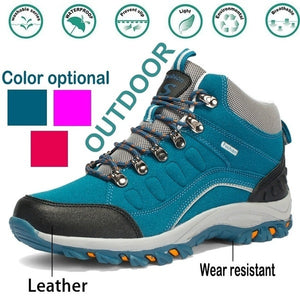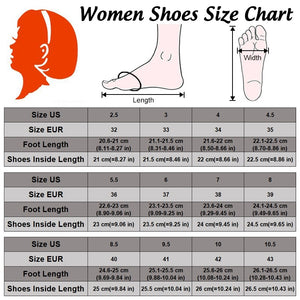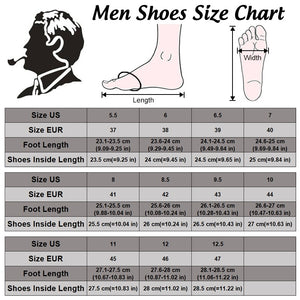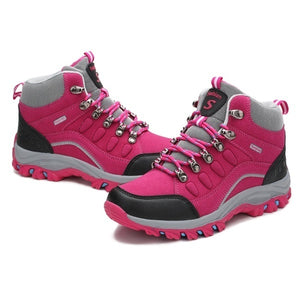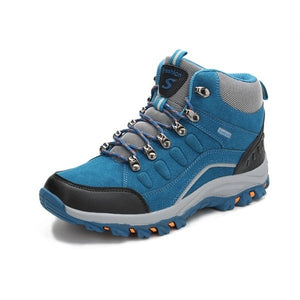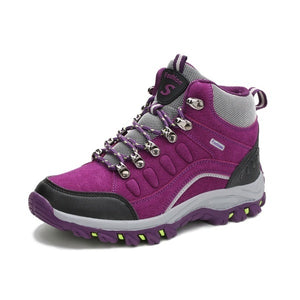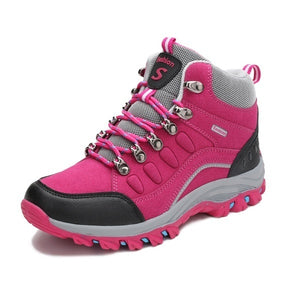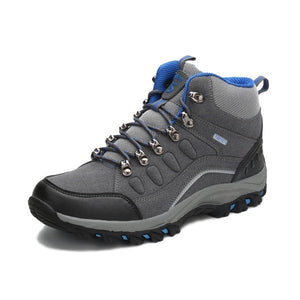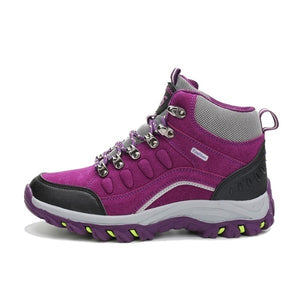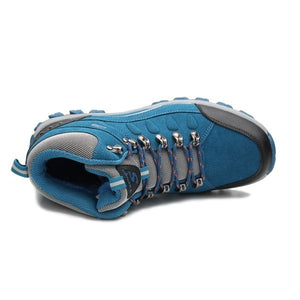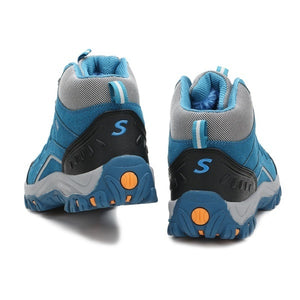# Plus Size 35-46 Mens \ Womens Outdoor Boots Hiking Boots Trekking Boots Mountaineering Shoes Climbing Boots

Regular price
\$11.09
Sale price
\$11.09
Regular price
\$596.00
Sold out
Unit price
per

Plus Size 35-46 Mens \ Womens Outdoor Boots Hiking Boots Trekking Boots Mountaineering Shoes Climbing Boots

Dear friends£º
Please select the color according to the color indicated in the picture.
pls choose size according to your foot length
Women Size:
Foot length range Shoes insole length US EUR
22.1-22.5cm(8.70¡«8.86 in) =22.5cm(=8.86 in) =4.5 =35
22.6-23cm(8.90¡«9.06 in) =23cm(=9.06 in) =5.5 =36
23.1-23.5cm(9.09¡«9.25 in) =23.5cm(=9.25 in) =6 =37
23.6-24cm(9.29¡«9.45 in) =24cm(=9.45 in) =7 =38
24.1-24.5cm(9.49¡«9.65in) =24.5cm(=9.65 in) =8 =39
24.6-25cm(9.69¡«9.84 in) =25cm(=9.84 in) =8.5 =40
25.1-25.5cm(9.88¡«10.04 in) =25.5cm(=10.04 in) =9.5 =41
25.6-26cm(10.08¡«10.24 in) =26cm(=10.24 in) =10 =42
26.1-26.5cm(10.28¡«10.43 in) =26.5cm(=10.43 in) =10.5 =43
26.6-27 cm(10.47-10.63in) =27cm(=10.63in) =11 =44
Notice:
1. Due to the different monitor and light effect, the actual color of the item might be slightly different from the color showed on the pictures.
2. Please allow 1-3cm measuring deviation due to manual measurement.

Men Size£º
Foot Length range Shoes insole Length US EUR
23.6-24cm(9.29-9.45in) =24cm(=9.45in) =6 =38
24.1-24.5cm(9.49-9.64in) =24.5cm(=9.65in) =6.5 =39
24.6-25cm(9.69-9.84in) =25cm(=9.84in) =7 =40
25.1-25.5cm(9.88-10.04in) =25.5cm(=10.04in) =8 =41
25.6-26 cm(10.08-10.24in) =26cm(=10.24in) =8.5 =42
26.1-26.5cm(10.28-10.43in) =26.5cm(=10.43in) =9.5 =43
26.6-27 cm(10.47-10.63in) =27cm(=10.63in) =10 =44
27.1-27.5cm(10.67-10.83in) =27.5cm(=10.83in) =11 =45
27.6-28cm(10.87-11.02in) =28cm(=11.02in) =12 =46
28.1-28.5cm(11.06-11.22in) =28.5cm(=11.22in) =12.5 =47
Notice:
1. Due to the different monitor and light effect, the actual color of the item might be slightly different from the color showed on the pictures.
2. Please allow 1-3cm measuring deviation due to manual measurement.
Contact us if you have any problem. We will solve it immediately.